The complex Exponential Function is the complex function that we can define as a power series and that extends the real Exponential function to complex valuesThis series converges everywhere in the complex plane.

In this page we try to show the geometric nature of the mappingThe Exponential Function verifiesThe Exponential Function es periodic with period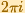.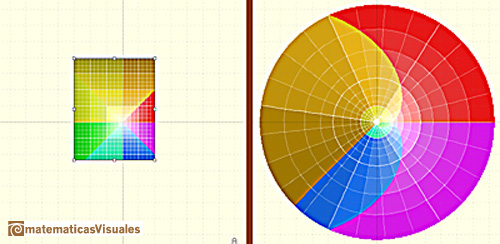The entiry w-plane (with the exception of the origin) will be filled by the image of any horizontal strip in the z-plante of height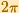.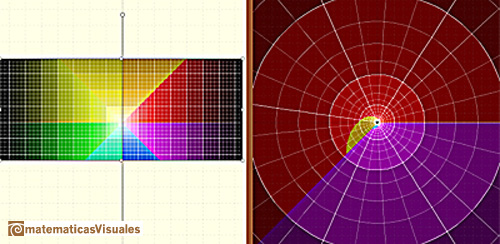A line is mapped to a spiral (or to a line or to a circle).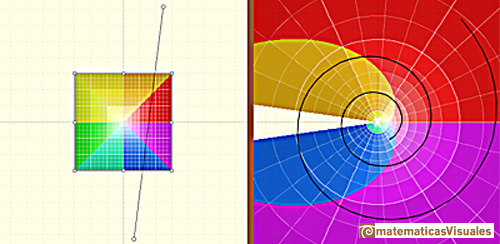Euler's formula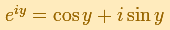can be interpreted as saying that the Exponential Function "wraps the imaginary axis round and round the unit circle like a piece of string" (Tristan Needham).The half-plane to the left of the imaginary axis is mapped to the interior of the unit circle, and the half-plane to the right of the imaginary axis is mapped to the exterior of the unit circle (Tristan Needham).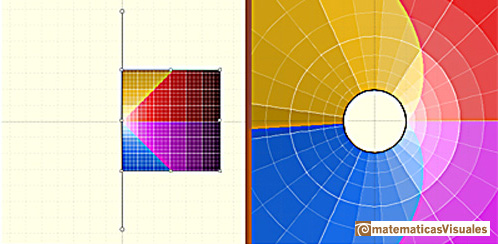The images of the small squares closely resemble squares and (related to this) any two intersecting lines map to curves that intersect at the same angle as the lines themselves (Tristan Needham).

REFERENCES

Tristan Needham - Visual Complex Analysis. (pags. 64-77) - Oxford University PressThe complex exponential function is periodic. His power series converges everywhere in the complex plane.The Complex Cosine Function extends the Real Cosine Function to the complex plane. It is a periodic function that shares several properties with his real ancestor.The Complex Cosine Function maps horizontal lines to confocal ellipses.After the definition of the natural logarithm function as an integral you can define the exponential function as the inverse function of the logarithm.We can study several properties of exponential functions, their derivatives and an introduction to the number e.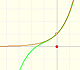By increasing the degree, Taylor polynomial approximates the exponential function more and more.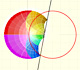Inversion is a plane transformation that transform straight lines and circles in straight lines and circles.The usual definition of a function is restrictive. We may broaden the definition of a function to allow f(z) to have many differente values for a single value of z. In this case f is called a many-valued function or a multifunction.Examples of complex functions: polynomials, rationals and Moebius Transformations.